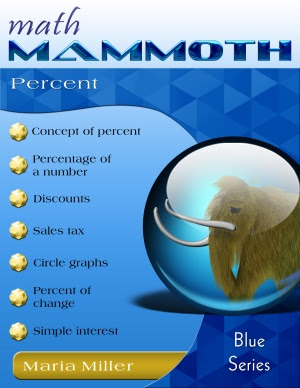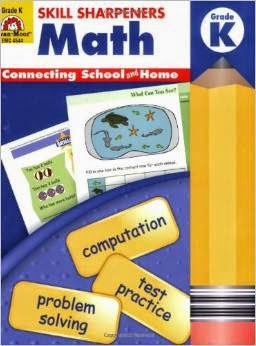## Posts

Showing posts from March, 2015

### Math Mammoth Percent book updatedMath Mammoth Percent is a worktext (with both instruction & exercises) that teaches students the concept of percent, how to calculate the percentage of a number, to figure discounts, sales tax, and interest, to draw circle graphs, to differentiate between a percent of change and a percent of comparison, and to know how to calculate both. The text is suitable for grades 6 through 8 (middle school). This book has been now updated to include many new lessons that will ALSO be in the upcoming Math Mammoth grade 7-B . This means that you can use it to continue pre-algebra studies after 7-A. See also these free sample pages (PDF): Contents and Introduction Review What Percentage...? Percentage of a Number Discounts Percent of Change --

### Kindergarten math placement test?